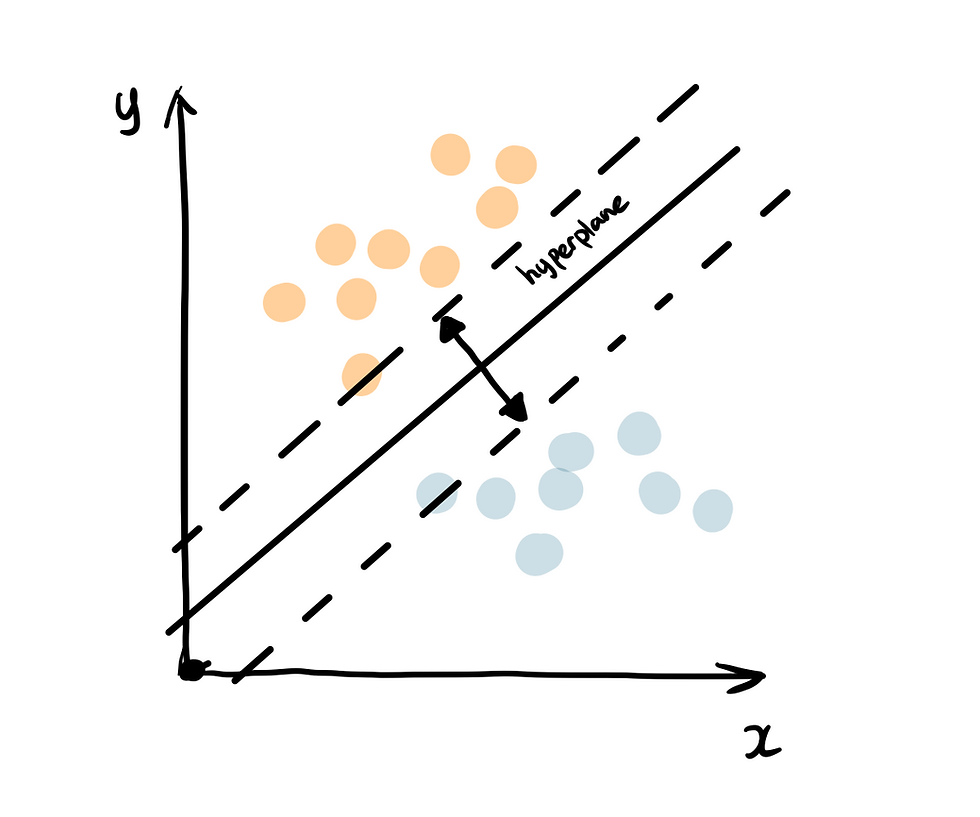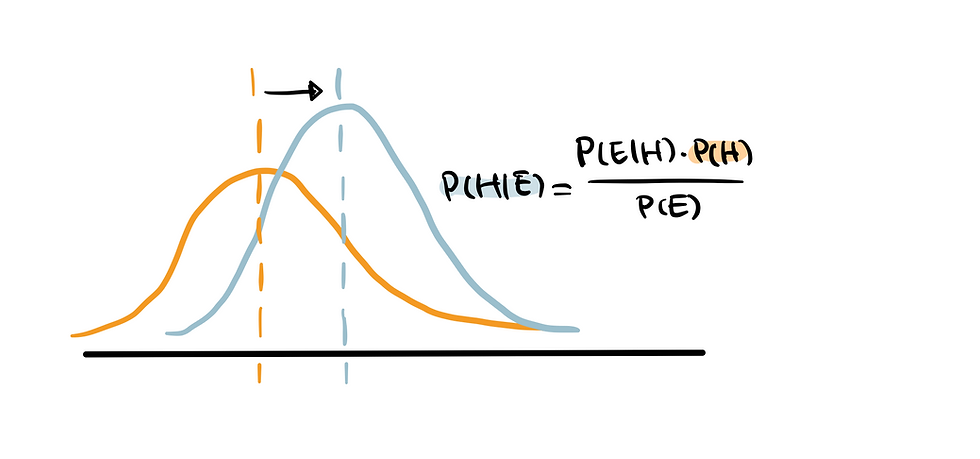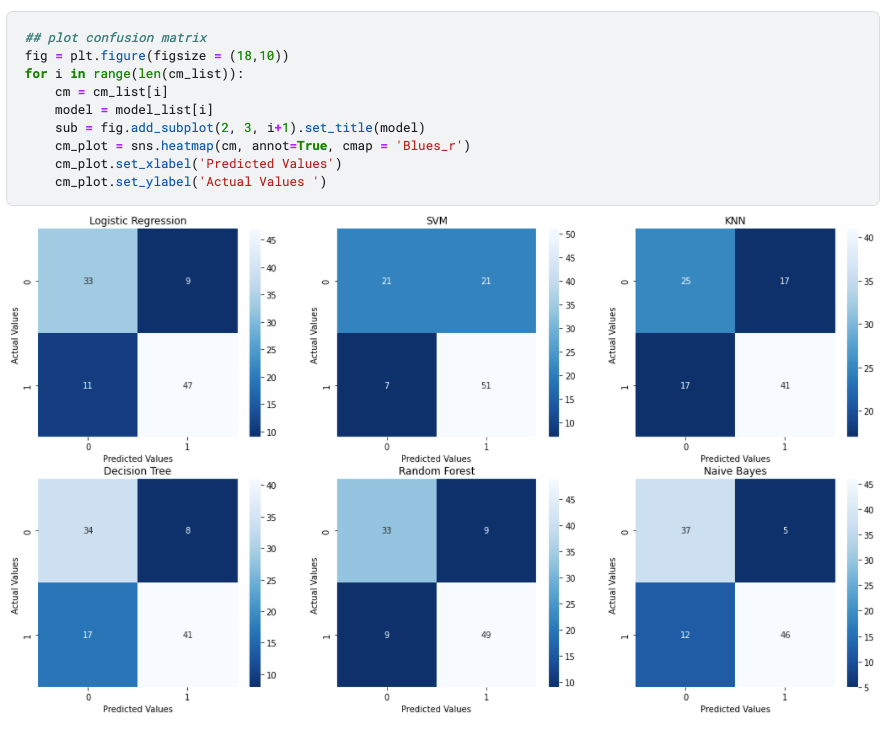top of pageSearch

# Top Machine Learning Algorithms for Classification

Updated: Mar 21, 2022## Types of Machine Learning Algorithms

### Supervised vs. Unsupervised vs. Reinforcement Learning

The easiest way to distinguish a supervised learning and unsupervised learning is to see whether the data is labelled or not.

Supervised learning learns a function to make prediction of a defined label based on the input data. It can be either classifying data into a category (classification problem) or forecasting an outcome (regression algorithms).

Unsupervised learning reveals the underlying pattern in the dataset that are not explicitly presented, which can be discovering the similarity of data points (clustering algorithms) or the hidden relationships of variables (association rule algorithms) ...

Reinforcement learning is another category of machine learning, where the agents learn to take actions based on its interaction with the environment, with the aim to maximize rewards. It is most similar to the learning process of human, following a trial-and-error method.

### Classification vs Regression

Supervised learning can be furthered categorized into classification and regression algorithms. Classification model identifies which category an object belongs to whereas regression model predicts a continuous output.

Sometimes there is an ambiguous line between classification algorithms and regression algorithms. Many algorithms can be used for both classification and regression, and classification is just regression model with a threshold applied. When the number is higher than the threshold it is classified as true while lower classified as false.

In this article, we will discuss top 6 machine learning algorithms for classification problems, including: logistic regression, decision tree, random forest, support vector machine, k-nearest neighbour and naive bayes. I will summarize the theory behind each as well as how to implement each using python. Check out the code for model pipeline here.

## 1. Logistic RegressionLogistics regression uses sigmoid function above to return the probability of a label. It is widely used when the classification problem is binary, for example true or false, win or lose, positive or negative etc.

The sigmoid function generates a probability output. And by comparing the probability with a pre-defined threshold, the object is assigned to a label accordingly. Check out my posts on logistic regression for a detailed walkthrough.

Below is the code snippet for a default logistic regression. I have also provided the common hyperparameters to experiment on, to see which combinations bring the best result.

```from sklearn.linear_model import LogisticRegression
dtc = DecisionTreeClassifier()
dtc.fit(X_train, y_train)
y_pred = dtc.predict(X_test)```

logistic regression common hyperparameters: penalty, max_iter, C, solver

## 2. Decision TreeDecision tree builds tree branches in a hierarchy approach and each branch can be considered as an if-else statement. The branches develop by partitioning the dataset into subsets, based on most important features. Final classification happens at the leaves of the decision tree.

```from sklearn.tree import DecisionTreeClassifier
reg = LogisticRegression()
reg.fit(X_train, y_train)
y_pred = reg.predict(X_test)```

decision tree common hyperparameters: criterion, max_depth, min_samples_split, min_samples_leaf; max_features

## 3. Random ForestAs the name suggest, random forest is a collection of decision trees. It is a common type of ensemble methods - which aggregate results from multiple predictors. Random forest additionally utilizes bagging technique that allows each tree trained on a random sampling of original dataset and takes the majority vote from trees. Compared to decision tree, it has better generalization but less interpretable because of more layers added to the model.

```from sklearn.ensemble import RandomForestClassifier
rfc = RandomForestClassifier()
rfc.fit(X_train, y_train)
y_pred = rfc.predict(X_test)```

random forest common hyperparameters: n_estimators, max_features, max_depth, min_samples_split, min_samples_leaf, boostrap

## 4. Support Vector Machine (SVM)Support vector machine finds the best way to classify the data based on the position in relation to a border between positive class and negative class. This border is known as the hyperplane which maximize the distance between data points from different classes. Similar to decision tree and random forest, support vector machine can be used in both classification and regression, SVC (support vector classifier) is chosen for classification problem.

```from sklearn.svm import SVC
svc = SVC()
svc.fit(X_train, y_train)
y_pred = svc.predict(X_test)```

support vector machine common hyperparameters: c, kernel, gamma

## 5. K-Nearest Neighbour (KNN)You can think of k nearest neighbour algorithm as representing each data point in a n dimensional space - which is defined by n features. And it calculates the distance between one point to another, then assign the label of unobserved data based on the labels of nearest observed data points. KNN can also be used for building recommendation system, check out my article on "Collaborative Filtering for Movie Recommendation".

```from sklearn.neighbors import KNeighborsClassifier
knn = KNeighborsClassifier()
knn.fit(X_train, y_train)
y_pred = knn.predict(X_test)```

KNN common hyperparameters: n_neighbors, weights, leaf_size, p

## 6. Naive BayesNaive Bayes is based on Bayes' Theorem - an approach to calculate conditional probability based on prior knowledge, and the naive assumption that each feature is independent to each other. The biggest advantage of Naive Bayes is that, while most machine learning algorithms rely on large amount of training data, it performs relatively well even when the training data size is small. Gaussian Naive Bayes is a type of Naive Bayes classifier that follows the normal distribution.

```from sklearn.naive_bayes import GaussianNB
gnb = KNeighborsClassifier()
gnb.fit(X_train, y_train)
y_pred = gnb.predict(X_test)```

gaussian naive bayes common hyperparameters: priors, var_smoothing

For those visual learners:

## Build a Classification Model Pipeline

I have implemented a pipeline to iterate through the default state of each classification algorithm.

I chose the popular dataset Heart Disease UCI on Kaggle for predicting the presence of heart disease based on several health related factors.Use df.info() to have a summarized view of dataset, including data type, missing data and number of records.### 2. Exploratory Data Analysis (EDA)

Histogram, grouped bar chart and box plot are suitable EDA techniques for classification machine learning algorithms. If you'd like a more comprehensive guide to EDA, please see my post "Semi-Automated Exploratory Data Analysis Process in Python"

Univariate AnalysisTo perform univariate analysis, histogram is used for all features. This is because all features have been encoded into numeric values in the dataset. This saves us the time for categorical encoding that usually happens during the feature engineering stage.

Categorical Features vs. Target - Grouped Bar ChartTo show how categorical value weigh in determining the target value, grouped bar chart is a straightforward representation. For example, sex = 1 and sex = 0 have distinctly distribution of target value, which indicates it is likely to contribute more to the prediction of target. Contrarily, if the target distribution is the same regardless of the categorical features, it means that they are not correlated.

Numerical Features vs. Target - Box PlotBox plot shows how the values of numerical features varies across target groups. For example, we can tell that “oldpeak” have distinct difference when target is 0 vs. target is 1, suggesting that it is an important predictor. However, ‘trestbps' and 'chol’ appear to be less outstanding, since the box plot distribution is similar across target groups.

### 3. Split Dataset into Training and Testing Set

Classification algorithm falls under the category of supervised learning, so dataset needs to be split into a subset for training and a subset for testing (sometime also a validation set). The model is trained on the training set and then examined using the testing set.

```from sklearn.model_selection import train_test_split
from sklearn import preprocessing

X = df.drop(['target'], axis=1)
y = df["target"]

X_train, X_test, y_train, y_test = train_test_split(X, y, test_size=0.33, random_state=42)```

### 4. Machine Learning Model Pipeline

In order to create a pipeline, I append all top 6 classification algorithms mentioned above into the model list and later will iterate through them to train, test, predict and evaluate.### 5. Model EvaluationHere is an abstraction explanation of commonly used evaluation methods for classification models - accuracy, ROC & AUC and confusion matrix. Each of the following metrics is worth diving deeper, feel free to visit my article on logistic regression for a more detailed illustration.

1. Accuracy

Accuracy is the most straightforward indicator of the model performance. It measure the percentage of accurate predictions.

2. ROC & AUCROC is the plot of false positive rate against true positive rate at various classification threshold. AUC is the area under the ROC curve, and higher AUC indicates better model performance.3. Confusion matrix

Confusion matrix indicates the actual values vs. predicted values and summarize the true negative, false positive, false negative and true positive values in a matrix format.

 ​True Negative False Positive False Negative True Positive

Then we can use seaborn to visualize the confusion matrix in a heatmap.Based on three evaluations methods above, random forests and naive bayes have the best performance whereas KNN is not doing well. However, this doesn't mean that random forests and naive bayes are superior algorithms. We can only say that they are more suitable for this dataset where the size is relatively smaller and data is not at the same scale.

Each algorithm has its own preference and require different data processing and feature engineering techniques, for example KNN is sensitive to features at difference scale and multicollinearity affects the result of logistic regression. Understanding the characteristics of each allows us to balance the trade-off and select the appropriate model based on the dataset.